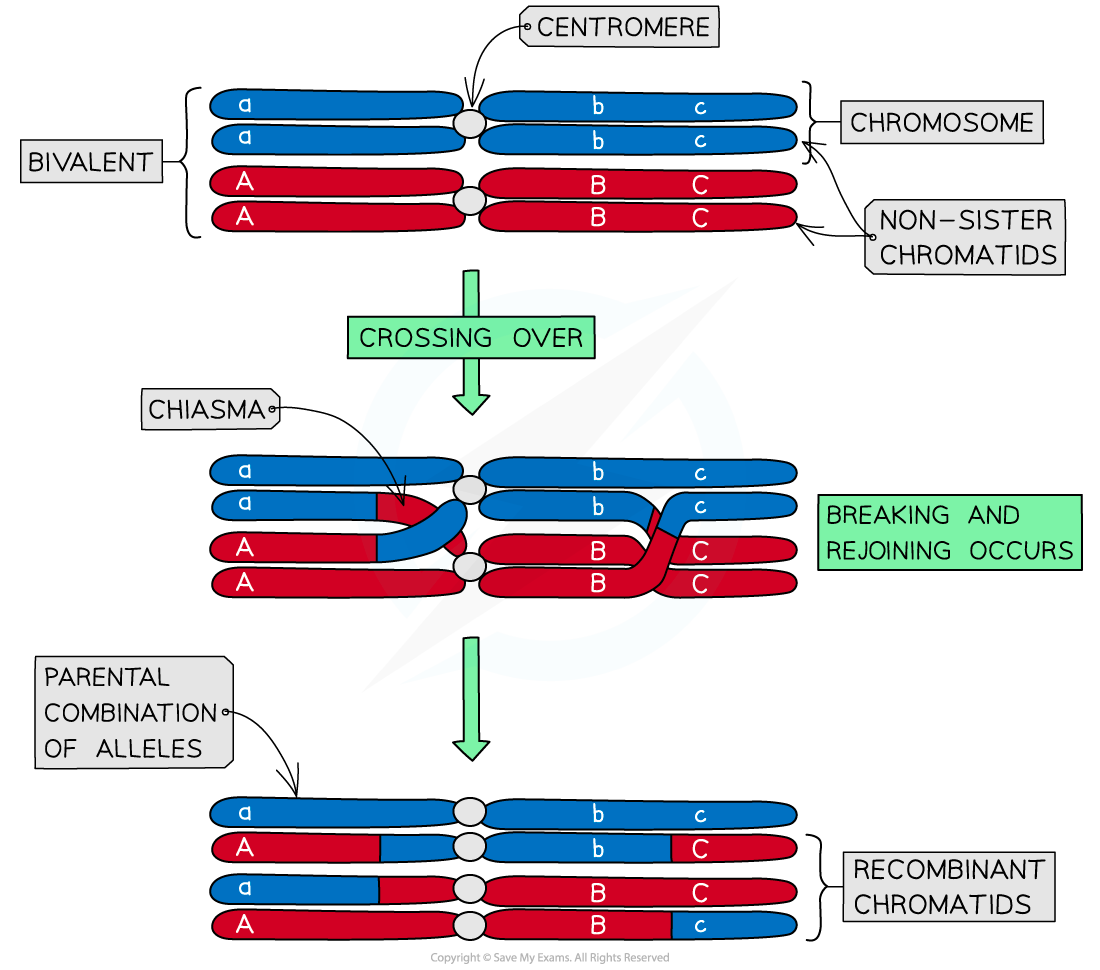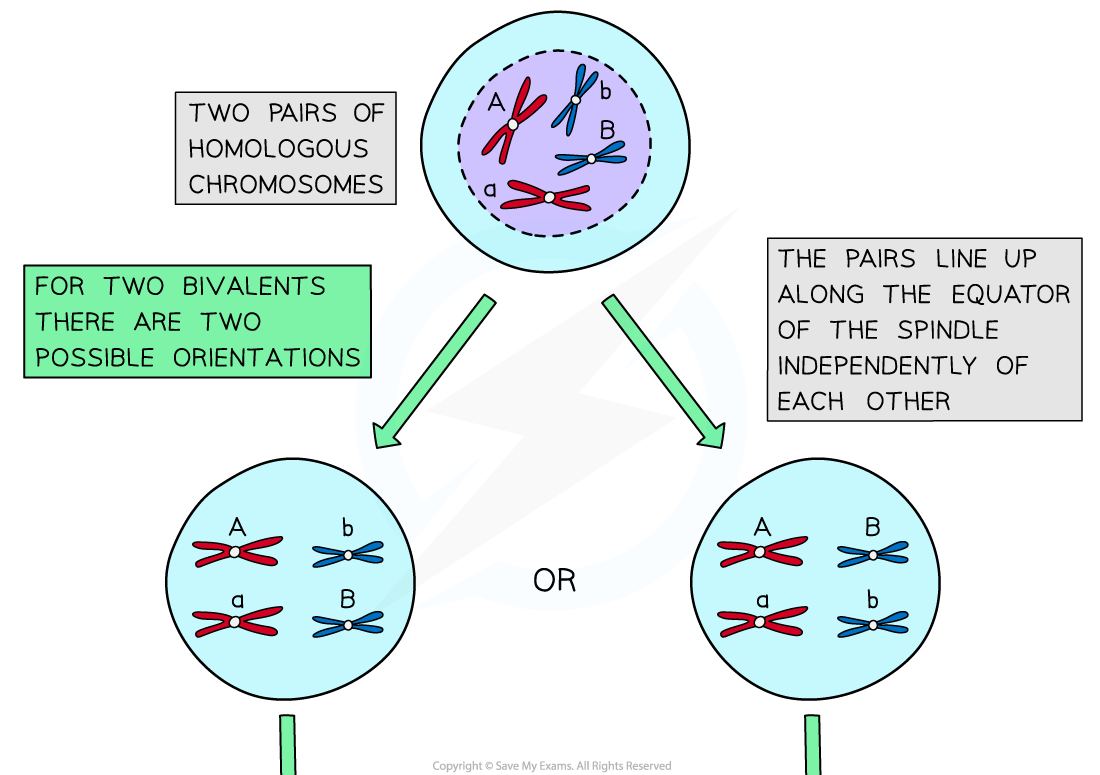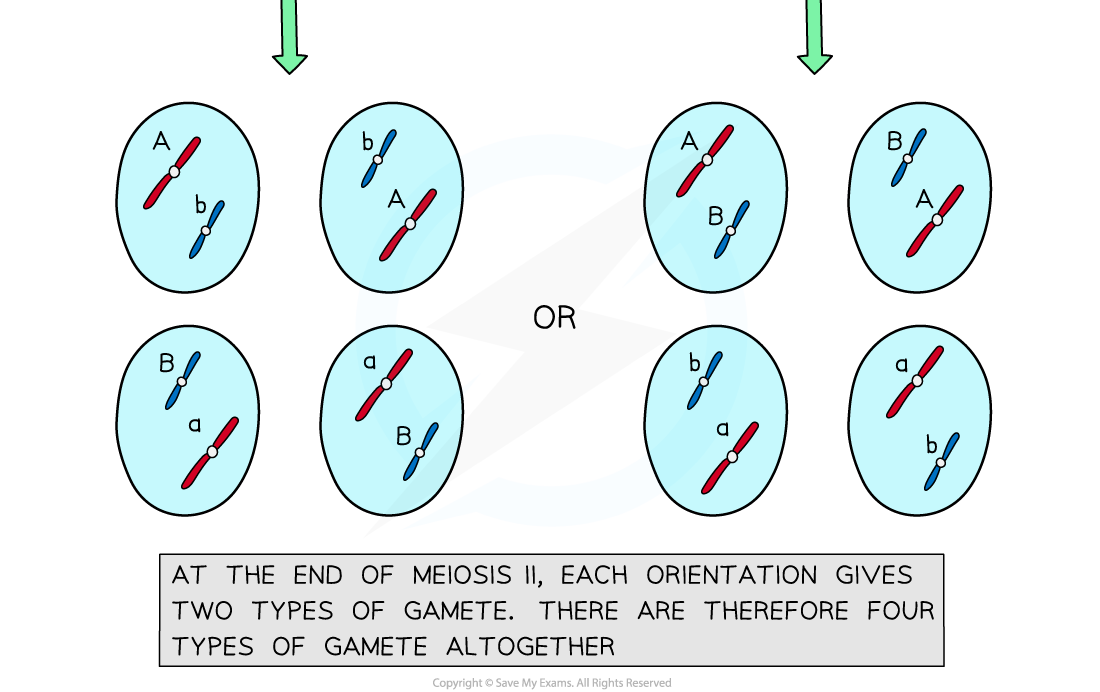# IB DP Biology: SL复习笔记3.2.3 Genetic Variation

### Genetic Variation & Meiosis

#### Crossing over and random orientation promote genetic variation

• Having genetically different offspring can be advantageous for natural selection and therefore increase the survival chances of a species
• Meiosis has several mechanisms that increase the genetic variation of gametes produced
• Both crossing over and random orientation result in different combinations of alleles in gametes

#### Crossing over

• Crossing over is the process by which non-sister chromatids exchange alleles
• Process:
• During prophase I of meiosis homologous chromosomes pair up and are in very close proximity to each other
• A pair of homologous chromosomes can be referred to as a bivalent
• At this point, there can be an exchange of genetic material (alleles) between non-sister chromatids in the bivalent
• The crossing points are called chiasmata
• This results in a new combination of alleles on the two chromosomes (these can be referred to as recombinant chromosomes)
• This swapping of alleles is a significant source of genetic variation because it can occur at multiple random positions along the chromosome
• Crossing over can happen anywhere along the chromosome but is more likely to occur further down the chromosome away from the centromereCrossing over occurring between two non-sister chromatids

#### Random orientation

• The random orientation of homologous pairs along the equator of the cell during metaphase I result in the production of different allele combinations in daughter cells
• In prophase I, homologous chromosomes pair up and in metaphase I, they align along the equator of the cell
• Each pair can be arranged with either chromosome on top, this is completely random
• The orientation of each homologous pair is random/independent (unaffected by the orientation of any other pair)
• In anaphase I the homologous chromosomes are separated and pulled apart to different poles
• The combination of alleles that end up in each daughter cell depends on how the pairs of homologous chromosomes were lined up
• The different combinations of chromosomes in daughter cells increases genetic variation between gametesThe random orientation of homologous chromosomes leads to different genetic combinations in daughter cells

#### The different combinations of chromosomes following meiosis

• The number of possible chromosomal combinations resulting from random assortment is equal to 2n
• n is the number of homologous chromosome pairs or haploid number
• For humans: the number of chromosomes is 46 meaning the number of homologous chromosome pairs is 23 so the calculation would be:
• 223 = 8,388,608 possible chromosomal combinations

#### Worked Example

Calculate how many different chromosomal combinations can result from meiosis in a plant species which has a diploid number of 16. Assume no crossing over occurs.[1 mark]

Step 1: Use the relevant formula

2n

Step 2: Calculate the haploid number

Diploid number (2n) = 16

Haploid number (n) = 16 ÷ 2 = 8

Step 3: Substitute in figures

28 = 256

There are 256 different chromosomal combinations that can occur.

### Genetic Variation & Fertilisation

#### Fusion of gametes from different parents promotes genetic variation

• Meiosis creates genetic variation between the gametes produced by an individual through crossing over and random orientation
• This means each gamete carries substantially different alleles
• During fertilisation, any male gamete can fuse with any female gamete to form a zygote
• This random fusion of gametes at fertilisation creates genetic variation between zygotes as each will have a unique combination of alleles

#### The different combinations of chromosomes following fertilisation

• In random fertilisation, any two gametes may fuse together
• Therefore the formula to calculate the number of combinations of chromosomes after the random fertilisation of two gametes is (2n)2
• n is the haploid number and 2 is the number of gametes
• Therefore in humans, when the haploid number is 23, the number of combinations following fertilization is (223)2­­  = 70,368,744,177,664
• This explains why relatives can differ so much from each other. Even with the same parents, individuals can be genetically distinct due to variation at the meiosis and fertilization stage (as well as other possible mutations and crossing-over)

#### Worked Example

Calculate the number of different possible chromosome combinations after the random fertilization of an ovule and pollen nuclei from the same plant species (Diploid number = 16).[2 marks]

Step 1: State formula for random fertilisation between any two gametes

(2n)2

Step 2: Use information from question to state haploid number

n = 8

Step 3: Substitute in figures

(2n)2

(28)2

#### Exam Tip

These sources of genetic variation explain why relatives can differ so much from each other. Even with the same parents, individuals can be genetically distinct due to the processes outlined above.While we can calculate the number of chromosomal combinations that result from random orientation and random fertilisation, the number of combinations from crossing over is infinite, or as good as!